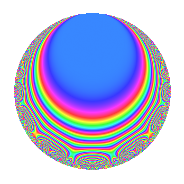# Properties

 Label 286.2.aLevel 286 Weight 2 Character orbit a Rep. character $$\chi_{286}(1,\cdot)$$ Character field $$\Q$$ Dimension 9 Newforms 7 Sturm bound 84 Trace bound 5

# Related objects

## Defining parameters

 Level: $$N$$ = $$286 = 2 \cdot 11 \cdot 13$$ Weight: $$k$$ = $$2$$ Character orbit: $$[\chi]$$ = 286.a (trivial) Character field: $$\Q$$ Newforms: $$7$$ Sturm bound: $$84$$ Trace bound: $$5$$ Distinguishing $$T_p$$: $$3$$, $$5$$

## Dimensions

The following table gives the dimensions of various subspaces of $$M_{2}(\Gamma_0(286))$$.

Total New Old
Modular forms 46 9 37
Cusp forms 39 9 30
Eisenstein series 7 0 7

The following table gives the dimensions of the cuspidal new subspaces with specified eigenvalues for the Atkin-Lehner operators and the Fricke involution.

$$2$$$$11$$$$13$$FrickeDim.
$$+$$$$+$$$$+$$$$+$$$$1$$
$$+$$$$+$$$$-$$$$-$$$$1$$
$$+$$$$-$$$$+$$$$-$$$$3$$
$$-$$$$+$$$$+$$$$-$$$$1$$
$$-$$$$+$$$$-$$$$+$$$$1$$
$$-$$$$-$$$$-$$$$-$$$$2$$
Plus space$$+$$$$2$$
Minus space$$-$$$$7$$

## Trace form

 $$9q$$ $$\mathstrut -\mathstrut q^{2}$$ $$\mathstrut +\mathstrut 9q^{4}$$ $$\mathstrut +\mathstrut 2q^{5}$$ $$\mathstrut +\mathstrut 4q^{6}$$ $$\mathstrut -\mathstrut 8q^{7}$$ $$\mathstrut -\mathstrut q^{8}$$ $$\mathstrut +\mathstrut 9q^{9}$$ $$\mathstrut +\mathstrut O(q^{10})$$ $$9q$$ $$\mathstrut -\mathstrut q^{2}$$ $$\mathstrut +\mathstrut 9q^{4}$$ $$\mathstrut +\mathstrut 2q^{5}$$ $$\mathstrut +\mathstrut 4q^{6}$$ $$\mathstrut -\mathstrut 8q^{7}$$ $$\mathstrut -\mathstrut q^{8}$$ $$\mathstrut +\mathstrut 9q^{9}$$ $$\mathstrut -\mathstrut 6q^{10}$$ $$\mathstrut +\mathstrut q^{11}$$ $$\mathstrut -\mathstrut q^{13}$$ $$\mathstrut +\mathstrut 8q^{15}$$ $$\mathstrut +\mathstrut 9q^{16}$$ $$\mathstrut +\mathstrut 14q^{17}$$ $$\mathstrut -\mathstrut 13q^{18}$$ $$\mathstrut -\mathstrut 12q^{19}$$ $$\mathstrut +\mathstrut 2q^{20}$$ $$\mathstrut +\mathstrut 8q^{21}$$ $$\mathstrut -\mathstrut q^{22}$$ $$\mathstrut -\mathstrut 4q^{23}$$ $$\mathstrut +\mathstrut 4q^{24}$$ $$\mathstrut -\mathstrut q^{25}$$ $$\mathstrut +\mathstrut 5q^{26}$$ $$\mathstrut +\mathstrut 12q^{27}$$ $$\mathstrut -\mathstrut 8q^{28}$$ $$\mathstrut +\mathstrut 10q^{29}$$ $$\mathstrut -\mathstrut 4q^{30}$$ $$\mathstrut -\mathstrut 4q^{31}$$ $$\mathstrut -\mathstrut q^{32}$$ $$\mathstrut +\mathstrut 4q^{33}$$ $$\mathstrut -\mathstrut 2q^{34}$$ $$\mathstrut +\mathstrut 28q^{35}$$ $$\mathstrut +\mathstrut 9q^{36}$$ $$\mathstrut -\mathstrut 6q^{37}$$ $$\mathstrut +\mathstrut 4q^{38}$$ $$\mathstrut -\mathstrut 4q^{39}$$ $$\mathstrut -\mathstrut 6q^{40}$$ $$\mathstrut -\mathstrut 2q^{41}$$ $$\mathstrut -\mathstrut 12q^{42}$$ $$\mathstrut -\mathstrut 12q^{43}$$ $$\mathstrut +\mathstrut q^{44}$$ $$\mathstrut -\mathstrut 14q^{45}$$ $$\mathstrut +\mathstrut 8q^{46}$$ $$\mathstrut -\mathstrut 20q^{47}$$ $$\mathstrut +\mathstrut 9q^{49}$$ $$\mathstrut -\mathstrut 15q^{50}$$ $$\mathstrut -\mathstrut 28q^{51}$$ $$\mathstrut -\mathstrut q^{52}$$ $$\mathstrut +\mathstrut 14q^{53}$$ $$\mathstrut -\mathstrut 8q^{54}$$ $$\mathstrut +\mathstrut 6q^{55}$$ $$\mathstrut -\mathstrut 24q^{57}$$ $$\mathstrut -\mathstrut 18q^{58}$$ $$\mathstrut +\mathstrut 12q^{59}$$ $$\mathstrut +\mathstrut 8q^{60}$$ $$\mathstrut -\mathstrut 30q^{61}$$ $$\mathstrut -\mathstrut 4q^{62}$$ $$\mathstrut -\mathstrut 48q^{63}$$ $$\mathstrut +\mathstrut 9q^{64}$$ $$\mathstrut +\mathstrut 2q^{65}$$ $$\mathstrut -\mathstrut 4q^{66}$$ $$\mathstrut -\mathstrut 20q^{67}$$ $$\mathstrut +\mathstrut 14q^{68}$$ $$\mathstrut -\mathstrut 32q^{69}$$ $$\mathstrut +\mathstrut 52q^{71}$$ $$\mathstrut -\mathstrut 13q^{72}$$ $$\mathstrut -\mathstrut 10q^{73}$$ $$\mathstrut -\mathstrut 14q^{74}$$ $$\mathstrut -\mathstrut 28q^{75}$$ $$\mathstrut -\mathstrut 12q^{76}$$ $$\mathstrut +\mathstrut 2q^{80}$$ $$\mathstrut +\mathstrut 17q^{81}$$ $$\mathstrut -\mathstrut 26q^{82}$$ $$\mathstrut +\mathstrut 12q^{83}$$ $$\mathstrut +\mathstrut 8q^{84}$$ $$\mathstrut -\mathstrut 36q^{85}$$ $$\mathstrut +\mathstrut 8q^{86}$$ $$\mathstrut -\mathstrut q^{88}$$ $$\mathstrut +\mathstrut 2q^{89}$$ $$\mathstrut +\mathstrut 22q^{90}$$ $$\mathstrut -\mathstrut 4q^{91}$$ $$\mathstrut -\mathstrut 4q^{92}$$ $$\mathstrut +\mathstrut 32q^{93}$$ $$\mathstrut +\mathstrut 8q^{94}$$ $$\mathstrut +\mathstrut 24q^{95}$$ $$\mathstrut +\mathstrut 4q^{96}$$ $$\mathstrut -\mathstrut 6q^{97}$$ $$\mathstrut +\mathstrut 23q^{98}$$ $$\mathstrut +\mathstrut 13q^{99}$$ $$\mathstrut +\mathstrut O(q^{100})$$

## Decomposition of $$S_{2}^{\mathrm{new}}(\Gamma_0(286))$$ into irreducible Hecke orbits

Label Dim. $$A$$ Field CM Traces A-L signs $q$-expansion
$$a_2$$ $$a_3$$ $$a_5$$ $$a_7$$ 2 11 13
286.2.a.a $$1$$ $$2.284$$ $$\Q$$ None $$-1$$ $$-2$$ $$3$$ $$-1$$ $$+$$ $$+$$ $$-$$ $$q-q^{2}-2q^{3}+q^{4}+3q^{5}+2q^{6}-q^{7}+\cdots$$
286.2.a.b $$1$$ $$2.284$$ $$\Q$$ None $$-1$$ $$-1$$ $$-1$$ $$1$$ $$+$$ $$+$$ $$+$$ $$q-q^{2}-q^{3}+q^{4}-q^{5}+q^{6}+q^{7}+\cdots$$
286.2.a.c $$1$$ $$2.284$$ $$\Q$$ None $$1$$ $$-1$$ $$-3$$ $$-5$$ $$-$$ $$+$$ $$-$$ $$q+q^{2}-q^{3}+q^{4}-3q^{5}-q^{6}-5q^{7}+\cdots$$
286.2.a.d $$1$$ $$2.284$$ $$\Q$$ None $$1$$ $$-1$$ $$1$$ $$3$$ $$-$$ $$-$$ $$-$$ $$q+q^{2}-q^{3}+q^{4}+q^{5}-q^{6}+3q^{7}+\cdots$$
286.2.a.e $$1$$ $$2.284$$ $$\Q$$ None $$1$$ $$2$$ $$-1$$ $$1$$ $$-$$ $$+$$ $$+$$ $$q+q^{2}+2q^{3}+q^{4}-q^{5}+2q^{6}+q^{7}+\cdots$$
286.2.a.f $$1$$ $$2.284$$ $$\Q$$ None $$1$$ $$2$$ $$1$$ $$-3$$ $$-$$ $$-$$ $$-$$ $$q+q^{2}+2q^{3}+q^{4}+q^{5}+2q^{6}-3q^{7}+\cdots$$
286.2.a.g $$3$$ $$2.284$$ 3.3.961.1 None $$-3$$ $$1$$ $$2$$ $$-4$$ $$+$$ $$-$$ $$+$$ $$q-q^{2}+\beta _{1}q^{3}+q^{4}+(\beta _{1}-\beta _{2})q^{5}+\cdots$$

## Decomposition of $$S_{2}^{\mathrm{old}}(\Gamma_0(286))$$ into lower level spaces

$$S_{2}^{\mathrm{old}}(\Gamma_0(286)) \cong$$ $$S_{2}^{\mathrm{new}}(\Gamma_0(11))$$$$^{\oplus 4}$$$$\oplus$$$$S_{2}^{\mathrm{new}}(\Gamma_0(26))$$$$^{\oplus 2}$$$$\oplus$$$$S_{2}^{\mathrm{new}}(\Gamma_0(143))$$$$^{\oplus 2}$$